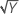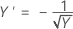# 随机批次的稳定性研究的方法

## 混合模型和对数似然

### 混合模型的一般形式

y =+ Z1μ1 + Z2μ2 + ... + Zcμc + ε

### 表示法

y响应值的 n x 1 向量
X固定效应的 n x p 设计矩阵，pn
Zi模型中第 i 个随机效应的 n x mi 设计矩阵
β未知参数的 p x 1 向量
μiN(0, σ2i) 中的自变量的 mi x 1 向量
εN(0, σ2i) 中的自变量的 n x 1 向量
c模型中的随机效应数

### 固定模型的特定形式

y =+ Z1μ1 + Z2μ2 + ε

y =+ Z1μ1 + ε

V(σ2) = V(σ2, σ21, ... , σ2c) = σ2In + σ21Z1Z'1 + ... + σ2cZcZ'c

σ2 = (σ2, σ21, ... , σ2c)'

σ2, σ21, ... , σ2c are called variance components.

V(σ2) = σ2H(θ) = σ2[In + θ1Z1Z'1 + ... + θcZcZ'c]

### 表示法

n观测值个数
pβ 中的参数个数，稳定性研究有 2 个参数
σ2误差方差分量
X设计矩阵 –– 对于固定项、常量和时间
H(θ)In + θ1Z1Z'1 + ... + θcZcZ'c
In具有 n 个行和列的恒等矩阵
θii 个随机项的方差与误差方差的比率
Zi模型中第 i 个随机效应的已知编码的 n x mi 矩阵
mii 个随机效应的水平数
c模型中的随机效应数
|H(θ)|H(θ) 的行列式
X'X 的转置
H-1(θ)H(θ)

## Box-Cox 变换

Box-Cox 变换选择能够最小化残差平方和的 lambda 值（如下所示）。由此生成的变换是 Yλ（当 λ ≠ 0 时）及 ln(Y)（当 λ = 0 时）。当 λ < 0 时，Minitab 还会将变换后响应乘以 −1，以维持未变换响应的顺序。

Minitab 搜索介于 −2 和 2 之间的最优值。此区间以外的值生成的拟合可能较差。

Lambda (λ) 值 变换
λ = 2 Y′ = Y 2
λ = .5 Y′ =λ = 0 Y′ = ln(Y )
λ = −.5λ = −1 Y′ = −1 / Y

## 随机批次模型选择

1. 时间 + 批次 + 批次*时间（批次的斜率和截距都不相等）
2. 时间 + 批次（批次的斜率相等，截距不相等）
3. 时间（批次的斜率和截距都相等）

### 模型 1 和模型 2 之间的检验

p = 0.5 * Prob(χ21 > 差分) + 0.5 * Prob(χ22 > 差分)

### 模型 2 和模型 3 之间的检验

p = 0.5 * Prob(χ21 > 差分)

### 表示法

La模型 a 的对数似然
p检验的 p 值
Prob(χ21> 差分)自由度为 1 的卡方分布中的随机变量的概率大于差分
Prob(χ22> 差分)自由度为 2 的卡方分布中的随机变量的概率大于差分

### 参考资料

1. Searle S.R.、Casella G. 和 McCuloch C.E. (1992)。Variance Components（方差分量）
2. West B.T.、Welch K.B. 和 Galecki A.T. (2007)。Linear Mixed Models: A Practical Guide Using Statistical Software（线性混合模型：使用统计软件的实用指南）。
3. Chow S. (2007)。Statistical Design and Analysis of Stability Studies（稳定性研究的统计设计和分析）。